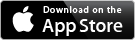Math Assist - Formula Reference Sheets

 Home uCalc Math Assist Record It! uDerivative About

 Math Assist : Ad supported Free in app storeMath Assist+ : No adds \$0.99 in app storeIt is hard to imagine surviving the rigor of college calculus and advanced engineering classes without a good set of math and calculus tables.

This iPhone application will supply you with everything you need through the first year (maybe two) of math. You will find the organization and flow will simplify your search.

The interface allows quick location of your formulas- including Algebra, Trigonometry, Derivatives, and Integrals. Each of these topics contains a single-click entry to a definitions to 5 to 10 pages of important formulas and information.

The current list of topics is includes more than 365 formulas (send suggestions to support@CreativeMindsetSoftware.com for more)-

Algebra

 Arithmetic Complex numbers Cubic formula Exponents Factoring Inequalities Logarithms Quadratic formula Radicals

Trigonometry

 Acute angles Addition formula Arbitrary angles Double angle formulas Fundamental identities Graphed inverse functions Graphed functions Half-angle formulas Real numbers Unit circle

Derivatives

 Critical and inflection points Definition of the derivative Exponential General rules Hyperbolic geometry Trigonometry

Integrals

 Basic forms Basic trigonometric forms Common integrals Common substitutions Exponent and logarithmic forms Fundamental integrals Green's Theorem Hyperbolic functions Indefinite integrals Reciprocals of trigonometric forms

For additional help or information contact support@CreativeMindsetSoftware.com